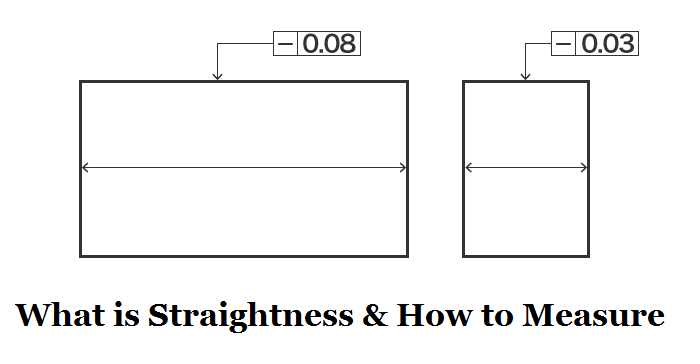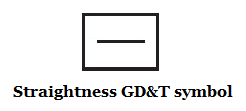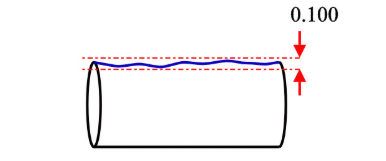> > Guide to Straightness – Straightness Definition, Measurement & Difference Between Flatness and Parallelism | CNCLATHING
> > Guide to Straightness – Straightness Definition, Measurement & Difference Between Flatness and Parallelism | CNCLATHING

# Guide to Straightness – Straightness Definition, Measurement & Difference Between Flatness and Parallelism | CNCLATHING

2020.10.9

Straightness is an important term to determine whether a CNC machining part conforms to the required specification. What is straightness and how to measure it? In this article, we outline the surface straightness definition, GD&T symbol, expression, measurement and difference between flatness and parallelism.## What is Straightness - How to Define Straightness

Straightness is a type of form tolerance introduced in GD&T, it can be defined as the extent to which a line element can deviate from its perfectness. To simply understand straightness, if we have a flat plate and the straightness applied to the top surface is 0.100, look at its edge view or cut any cross-section along with its length, the upper line of the cross-section represents the top surface intersects this section plane, that line cannot deviate from perfect straightness by more than the tolerance. The standard form of straightness is a 2-dimensional tolerance that used to ensure the surface of a part is uniform, there is also axis straightness used to control the central axis of a part.Set an imaginary boundary as below, it represents the tolerance zone and the size of that is going to be one hundred thousand of an inch, which is expressed by the value in the tolerance frame. The line represents the intersection of the top surface of the plate and the section plane can be any shape within this tolerance boundary.## How to Measure Straightness

Comparing these two measurement methods, the coordinate measuring machine is more versatile and accurate, while it should take lots of expenses, using a height gauge has more limitations but it’s much cheaper.

1. Use gauge measures along a straight line to check how flat or straight the line is along this surface through measuring the height variation. Ensure the object to be measured is fixed so that the height is evenly matched on the left and right. The difference between the maximum and minimum values is straightness.

2. Use a coordinate measuring machine (CMM) to measure the straightness as long as the operator puts the stylus lightly on the object to be measured. CMM machines can also be used to measure the part that can’t be secured level (a height gauge can’t do that).

## Difference Between Straightness and Flatness

1. Flatness is a property of a plane, while straightness is the profile of a line.

2. Straightness can be regarded as the 2D version of flatness.

3. Flatness measures the variance across a 2-dimensional plane, straightness only measures the variance on a straight line.

4. A flat plane does not vary vertically but can be curvy, a straight line does not vary left or right.

## Difference Between Straightness and Parallelism

1. There is a datum for parallelism measurement, while the measurement of straightness is no reference.

2. Parallelism is the distance between two parallel planes compared to the datum. Straightness is the distance between the highest point and the lowest point on a straight line.